• ## 平衡二叉树

万次阅读 多人点赞 2018-08-15 17:12:47
一、AVL树简介 AVL树的名字来源于它的发明作者G.M....平衡二叉树定义(AVL)：它或者是一颗空树，或者具有以下性质的二叉排序树：它的左子树和右子树的深度之差(平衡因子)的绝对值不超过1，且它的左子树和右子...
一、AVL树简介

AVL树的名字来源于它的发明作者G.M. Adelson-Velsky 和 E.M. Landis。AVL树是最先发明的自平衡二叉查找树（Self-Balancing Binary Search Tree,简称平衡二叉树）。
平衡二叉树定义(AVL)：它或者是一颗空树，或者具有以下性质的二叉排序树：它的左子树和右子树的深度之差(平衡因子)的绝对值不超过1，且它的左子树和右子树都是一颗平衡二叉树。

一棵AVL树有如下必要条件：
条件一：它必须是二叉查找树。条件二：每个节点的左子树和右子树的高度差至多为1。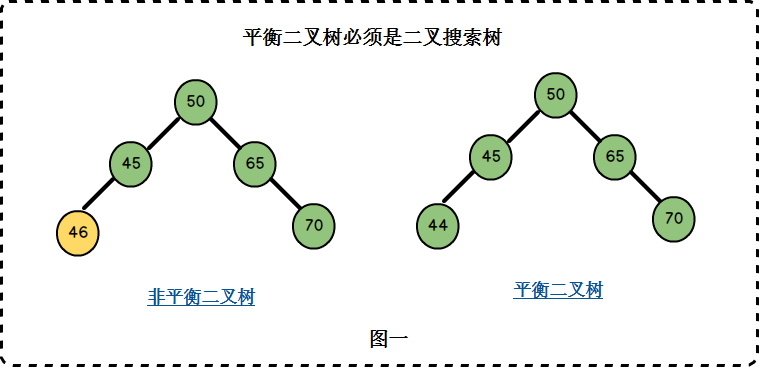图一中左边二叉树的节点45的左孩子46比45大，不满足二叉搜索树的条件，因此它也不是一棵平衡二叉树。 右边二叉树满足二叉搜索树的条件，同时它满足条件二，因此它是一棵平衡二叉树。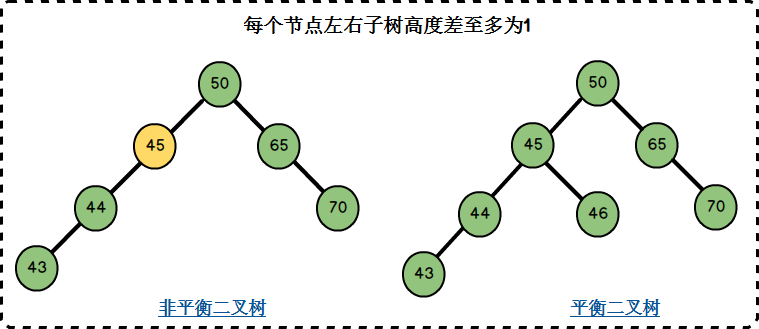左边二叉树的节点45左子树高度2，右子树高度0，左右子树高度差为2-0=2，不满足条件二； 右边二叉树的节点均满足左右子树高度差至多为1，同时它满足二叉搜索树的要求，因此它是一棵平衡二叉树。
AVL树的查找、插入、删除操作在平均和最坏的情况下都是O（logn），这得益于它时刻维护着二叉树的平衡。如果我们需要查找的集合本身没有顺序，在频繁查找的同时也经常的插入和删除，AVL树是不错的选择。不平衡的二叉查找树在查找时的效率是很低的，因此，AVL如何维护二叉树的平衡是我们的学习重点。

二、AVL树相关概念
1. 平衡因子：将二叉树上节点的左子树高度减去右子树高度的值称为该节点的平衡因子BF(Balance Factor)。     在图二右边的AVL树上：     节点50的左子树高度为3，右子树高度为2，BF= 3-2 = 1；     节点45的左子树高度为2，右子树高度为1，BF= 2-1 = 1；     节点46的左子树高度为0，右子树高度为0，BF= 0-0 = 0；     节点65的左子树高度为0，右子树高度为1，BF= 0-1 = -1；     对于平衡二叉树，BF的取值范围为[-1,1]。如果发现某个节点的BF值不在此范围，则需要对树进行调整。
2. 最小不平衡子树：距离插入节点最近的，且平衡因子的绝对值大于1的节点为根的子树.。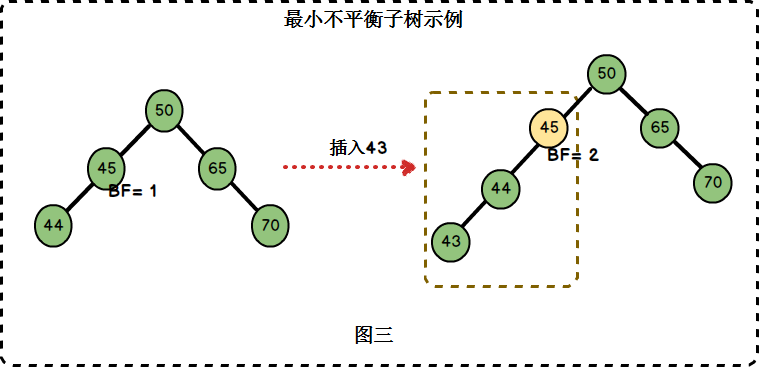在图三中，左边二叉树的节点45的BF = 1，插入节点43后，节点45的BF = 2。节点45是距离插入点43最近的BF不在[-1,1]范围内的节点，因此以节点45为根的子树为最小不平衡子树。

三、AVL树的平衡调整
定义平衡二叉树节点结构：
typedef struct Node
{
int key;
struct Node *left;
struct Node *right;
int height;
}BTNode;

整个实现过程是通过在一棵平衡二叉树中依次插入元素(按照二叉排序树的方式)，若出现不平衡，则要根据新插入的结点与最低不平衡结点的位置关系进行相应的调整。分为LL型、RR型、LR型和RL型4种类型，各调整方法如下(下面用A表示最低不平衡结点)：
1. LL型调整：

由于在A的左孩子(L)的左子树(L)上插入新结点，使原来平衡二叉树变得不平衡，此时A的平衡因子由1增至2。下面图1是LL型的最简单形式。显然，按照大小关系，结点B应作为新的根结点，其余两个节点分别作为左右孩子节点才能平衡，A结点就好像是绕结点B顺时针旋转一样。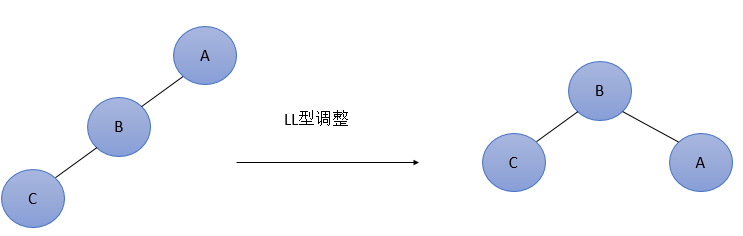LL型调整的一般形式如下图2所示，表示在A的左孩子B的左子树BL(不一定为空)中插入结点(图中阴影部分所示)而导致不平衡( h 表示子树的深度)。这种情况调整如下：①将A的左孩子B提升为新的根结点；②将原来的根结点A降为B的右孩子；③各子树按大小关系连接(BL和AR不变，BR调整为A的左子树)。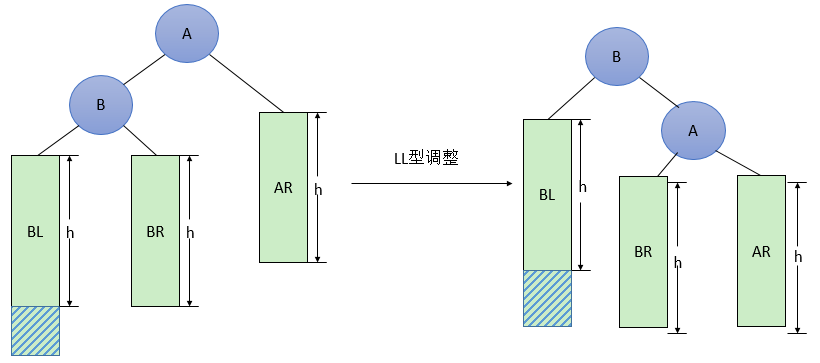图2  一般形式的LL型调整
代码实现：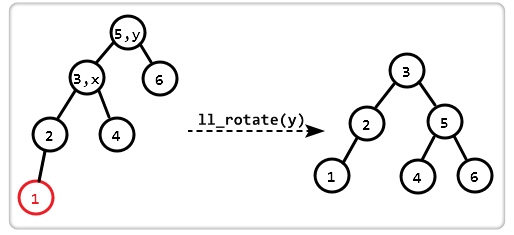BTNode *ll_rotate(BTNode *y)
{
BTNode *x = y->left;
y->left = x->right;
x->right = y;

y->height = max(height(y->left), height(y->right)) + 1;
x->height = max(height(x->left), height(x->right)) + 1;

return x;
}

2. RR型调整：

由于在A的右孩子(R)的右子树(R)上插入新结点，使原来平衡二叉树变得不平衡，此时A的平衡因子由-1变为-2。图3是RR型的最简单形式。显然，按照大小关系，结点B应作为新的根结点，其余两个节点分别作为左右孩子节点才能平衡，A结点就好像是绕结点B逆时针旋转一样。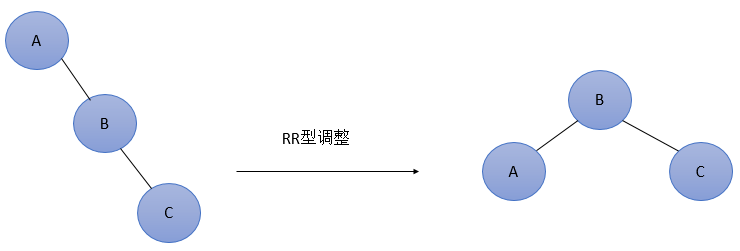RR型调整的一般形式如下图4所示，表示在A的右孩子B的右子树BR(不一定为空)中插入结点(图中阴影部分所示)而导致不平衡( h 表示子树的深度)。这种情况调整如下：
将A的右孩子B提升为新的根结点；将原来的根结点A降为B的左孩子各子树按大小关系连接(AL和BR不变，BL调整为A的右子树)。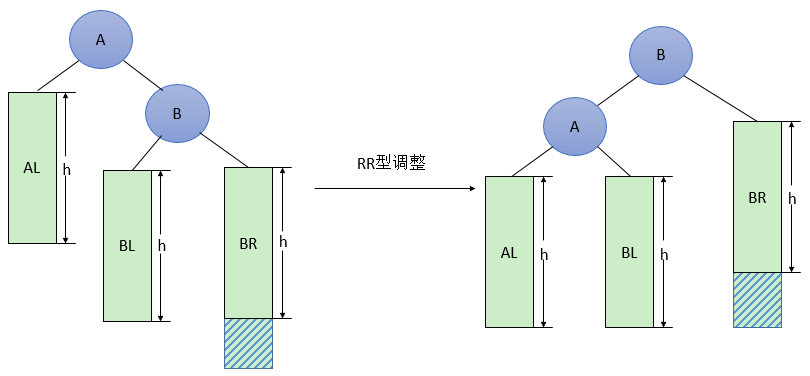图4  一般形式的RR型调整
代码实现：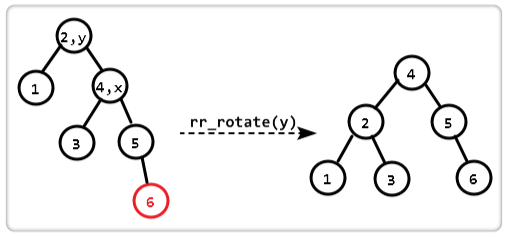BTNode *rr_rotate(struct Node *y)
{
BTNode *x = y->right;
y->right = x->left;
x->left = y;

y->height = max(height(y->left), height(y->right)) + 1;
x->height = max(height(x->left), height(x->right)) + 1;

return x;
}

3. LR型调整

由于在A的左孩子(L)的右子树(R)上插入新结点，使原来平衡二叉树变得不平衡，此时A的平衡因子由1变为2。图5是LR型的最简单形式。显然，按照大小关系，结点C应作为新的根结点，其余两个节点分别作为左右孩子节点才能平衡。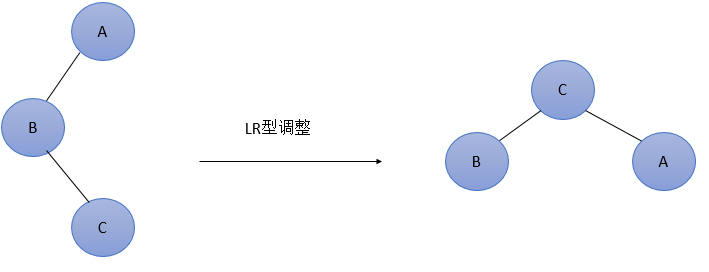图5  最简单的LR型调整

LR型调整的一般形式如下图6所示，表示在A的左孩子B的右子树(根结点为C，不一定为空)中插入结点(图中两个阴影部分之一)而导致不平衡( h 表示子树的深度)。这种情况调整如下：①将B的左孩子C提升为新的根结点；②将原来的根结点A降为C的右孩子；③各子树按大小关系连接(BL和AR不变，CL和CR分别调整为B的右子树和A的左子树)。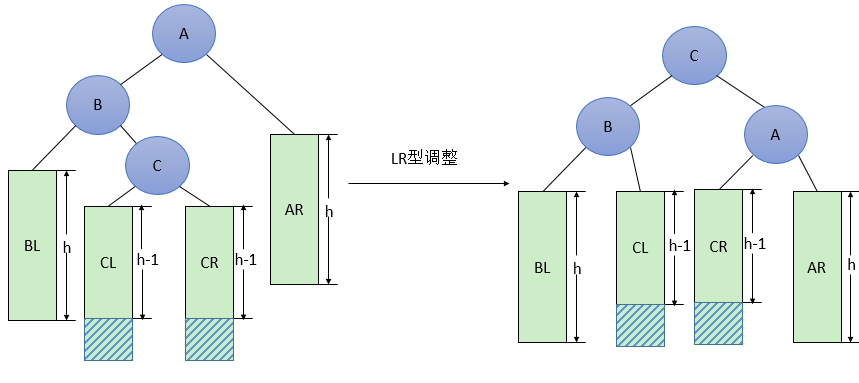图6  一般形式的LR型调整
3.1 代码实现：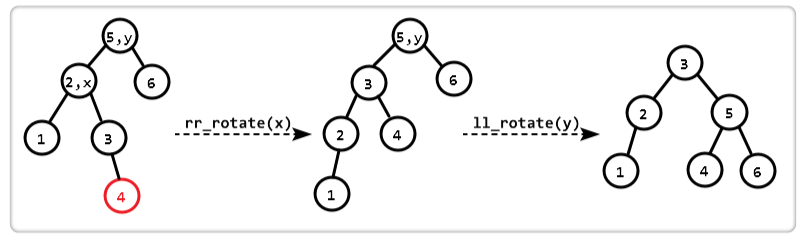BTNode* lr_rotate(BTNode* y)
{
BTNode* x = y->left;
y->left = rr_rotate(x);
return ll_rotate(y);
}

4. RL型调整：
由于在A的右孩子(R)的左子树(L)上插入新结点，使原来平衡二叉树变得不平衡，此时A的平衡因子由-1变为-2。图7是RL型的最简单形式。显然，按照大小关系，结点C应作为新的根结点，其余两个节点分别作为左右孩子节点才能平衡。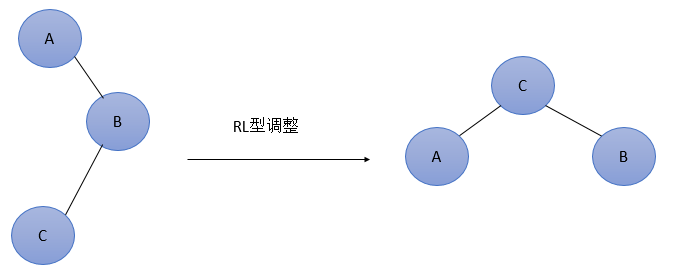图7  最简单的RL型调整
RL型调整的一般形式如下图8所示，表示在A的右孩子B的左子树(根结点为C，不一定为空)中插入结点(图中两个阴影部分之一)而导致不平衡( h 表示子树的深度)。这种情况调整如下：①将B的左孩子C提升为新的根结点；②将原来的根结点A降为C的左孩子；③各子树按大小关系连接(AL和BR不变，CL和CR分别调整为A的右子树和B的左子树)。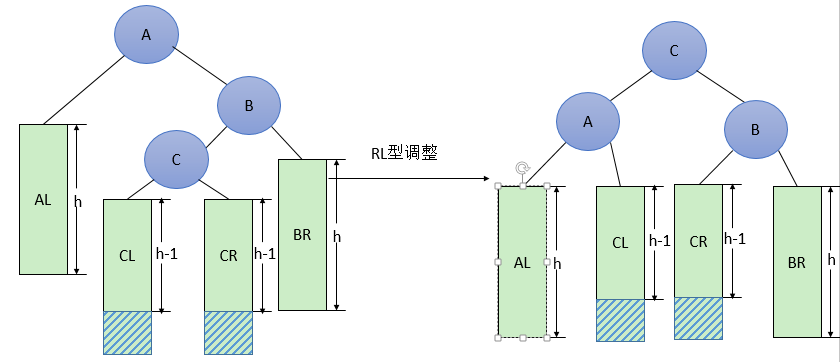图8  一般形式的RL型调整
4.1 代码实现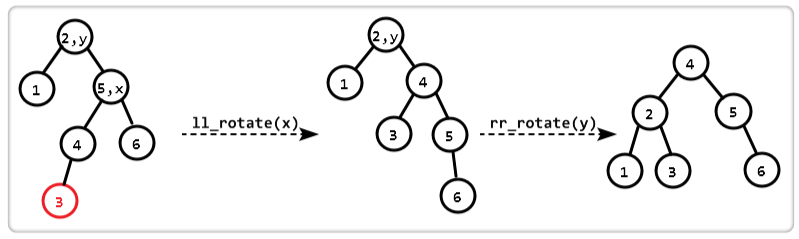Node* rl_rotate(Node* y)
{
Node * x = y->right;
y->right = ll_rotate(x);
return rr_rotate(y);
}

平衡二叉树实现的实例
选取一组数据分别为2，1，0，3，4，5，6，9，8，7的10个结点来构造平衡二叉树。
首先数据为2的结点作为根结点插入，接着插入1，仍是平衡的，再插入0是，2的平衡因子变为2，此时出现了不平衡，因此需要进行调整，最低不平衡结点为2，属于LL型，调整过程如图1所示。
图1

接着插入3，是平衡的，再插入4，此时出现了不平衡，结点 1 和 2 的平衡因子都为 -2，结点2为最低不平衡结点，属于RR型，调整过程如图2所示
图2

接着插入5，此时结点 1 的平衡因子为 -2，导致不平衡，结点1为最低不平衡结点，属于RR型，调整如图3所示。
图3

接着插入6，此时结点4的平衡因子为 -2，导致不平衡，结点4为最低不平衡结点，属于RR型，调整如图4所示。
图4

接着插入9，是平衡的，再插入8，此时结点 3、5、6 的平衡因子都为 -2，导致不平衡，结点6为最低不平衡结点，属于RL型，调整如图5所示。
图五

插入7，此时结点3、5的平衡因子为 -2，导致不平衡，最低不平衡结点为5，属于RL型，调整如图6所示。
图六

插入操作完整代码
#include<stdio.h>
#include<stdlib.h>

typedef struct Node
{
int key;
struct Node *left;
struct Node *right;
int height;
}BTNode;

int max(int a, int b);

int height(struct Node *N)
{
if (N == NULL)
return 0;
return N->height;
}

int max(int a, int b)
{
return (a > b) ? a : b;
}

BTNode* newNode(int key)
{
struct Node* node = (BTNode*)malloc(sizeof(struct Node));
node->key = key;
node->left = NULL;
node->right = NULL;
node->height = 1;
return(node);
}

BTNode* ll_rotate(BTNode* y)
{
BTNode *x = y->left;
y->left = x->right;
x->right = y;

y->height = max(height(y->left), height(y->right)) + 1;
x->height = max(height(x->left), height(x->right)) + 1;

return x;
}

BTNode* rr_rotate(BTNode* y)
{
BTNode *x = y->right;
y->right = x->left;
x->left = y;

y->height = max(height(y->left), height(y->right)) + 1;
x->height = max(height(x->left), height(x->right)) + 1;

return x;
}

int getBalance(BTNode* N)
{
if (N == NULL)
return 0;
return height(N->left) - height(N->right);
}

BTNode* insert(BTNode* node, int key)
{

if (node == NULL)
return newNode(key);

if (key < node->key)
node->left = insert(node->left, key);
else if (key > node->key)
node->right = insert(node->right, key);
else
return node;

node->height = 1 + max(height(node->left), height(node->right));

int balance = getBalance(node);

if (balance > 1 && key < node->left->key) //LL型
return ll_rotate(node);

if (balance < -1 && key > node->right->key)     //RR型
return rr_rotate(node);

if (balance > 1 && key > node->left->key)     //LR型
{
node->left = rr_rotate(node->left);
return ll_rotate(node);
}

if (balance < -1 && key < node->right->key)     //RL型
{
node->right = ll_rotate(node->right);
return rr_rotate(node);
}

return node;
}

void preOrder(struct Node *root)
{
if (root != NULL)
{
printf("%d ", root->key);
preOrder(root->left);
preOrder(root->right);
}
}

int main()
{
BTNode *root = NULL;

root = insert(root, 2);
root = insert(root, 1);
root = insert(root, 0);
root = insert(root, 3);
root = insert(root, 4);
root = insert(root, 4);
root = insert(root, 5);
root = insert(root, 6);
root = insert(root, 9);
root = insert(root, 8);
root = insert(root, 7);

printf("前序遍历：");
preOrder(root);
return 0;
}

删除代码：
struct Node * minValueNode(BTNode* node)
{
BTNode* current = node;

while (current->left != NULL)
current = current->left;

return current;
}

BTNode* deleteNode(BTNode* root, int key)
{

if (root == NULL)
return root;

if ( key < root->key )
root->left = deleteNode(root->left, key);

else if( key > root->key )
root->right = deleteNode(root->right, key);

else
{
if( (root->left == NULL) || (root->right == NULL) )
{
BTNode* temp = root->left ? root->left :  root->right;

if (temp == NULL)
{
temp = root;
root = NULL;
}
else
*root = *temp;
free(temp);
}
else
{
BTNode* temp = minValueNode(root->right);

root->key = temp->key;

root->right = deleteNode(root->right, temp->key);
}
}

if (root == NULL)
return root;

root->height = 1 + max(height(root->left), height(root->right));

int balance = getBalance(root);

if (balance > 1 && getBalance(root->left) >= 0) //LL型
return ll_rotate(root);

if (balance > 1 && getBalance(root->left) < 0) //LR型
{
root->left =  rr_rotate(root->left);
return ll_rotate(root);
}

if (balance < -1 && getBalance(root->right) <= 0) //RR型
return rr_rotate(root);

if (balance < -1 && getBalance(root->right) > 0)  //Rl型
{
root->right = ll_rotate(root->right);
return rr_rotate(root);
}

return root;
}


完整代码：
#include<stdio.h>
#include<stdlib.h>

typedef struct Node
{
int key;
struct Node *left;
struct Node *right;
int height;
}BTNode;

int max(int a, int b);

int height(struct Node *N)
{
if (N == NULL)
return 0;
return N->height;
}

int max(int a, int b)
{
return (a > b) ? a : b;
}

BTNode* newNode(int key)
{
struct Node* node = (BTNode*)malloc(sizeof(struct Node));
node->key = key;
node->left = NULL;
node->right = NULL;
node->height = 1;
return(node);
}

BTNode* ll_rotate(BTNode* y)
{
BTNode *x = y->left;
y->left = x->right;
x->right = y;

y->height = max(height(y->left), height(y->right)) + 1;
x->height = max(height(x->left), height(x->right)) + 1;

return x;
}

BTNode* rr_rotate(BTNode* y)
{
BTNode *x = y->right;
y->right = x->left;
x->left = y;

y->height = max(height(y->left), height(y->right)) + 1;
x->height = max(height(x->left), height(x->right)) + 1;

return x;
}

int getBalance(BTNode* N)
{
if (N == NULL)
return 0;
return height(N->left) - height(N->right);
}

BTNode* insert(BTNode* node, int key)
{

if (node == NULL)
return newNode(key);

if (key < node->key)
node->left = insert(node->left, key);
else if (key > node->key)
node->right = insert(node->right, key);
else
return node;

node->height = 1 + max(height(node->left), height(node->right));

int balance = getBalance(node);

if (balance > 1 && key < node->left->key) //LL型
return ll_rotate(node);

if (balance < -1 && key > node->right->key)     //RR型
return rr_rotate(node);

if (balance > 1 && key > node->left->key)     //LR型
{
node->left = rr_rotate(node->left);
return ll_rotate(node);
}

if (balance < -1 && key < node->right->key)     //RL型
{
node->right = ll_rotate(node->right);
return rr_rotate(node);
}

return node;
}

BTNode * minValueNode(BTNode* node)
{
BTNode* current = node;

while (current->left != NULL)
current = current->left;

return current;
}

BTNode* deleteNode(BTNode* root, int key)
{

if (root == NULL)
return root;

if (key < root->key)
root->left = deleteNode(root->left, key);

else if (key > root->key)
root->right = deleteNode(root->right, key);

else
{
if ((root->left == NULL) || (root->right == NULL))
{
BTNode* temp = root->left ? root->left : root->right;

if (temp == NULL)
{
temp = root;
root = NULL;
}
else
*root = *temp;
free(temp);
}
else
{
BTNode* temp = minValueNode(root->right);

root->key = temp->key;

root->right = deleteNode(root->right, temp->key);
}
}

if (root == NULL)
return root;

root->height = 1 + max(height(root->left), height(root->right));

int balance = getBalance(root);

if (balance > 1 && getBalance(root->left) >= 0) //LL型
return ll_rotate(root);

if (balance > 1 && getBalance(root->left) < 0) //LR型
{
root->left = rr_rotate(root->left);
return ll_rotate(root);
}

if (balance < -1 && getBalance(root->right) <= 0) //RR型
return rr_rotate(root);

if (balance < -1 && getBalance(root->right) > 0)  //Rl型
{
root->right = ll_rotate(root->right);
return rr_rotate(root);
}

return root;
}

void preOrder(struct Node *root)
{
if (root != NULL)
{
printf("%d ", root->key);
preOrder(root->left);
preOrder(root->right);
}
}

int main()
{
BTNode *root = NULL;

root = insert(root, 9);
root = insert(root, 5);
root = insert(root, 10);
root = insert(root, 0);
root = insert(root, 6);
root = insert(root, 11);
root = insert(root, -1);
root = insert(root, 1);
root = insert(root, 2);
printf("前序遍历：\n");
preOrder(root);

/* The constructed AVL Tree would be
9
/  \
1    10
/  \     \
0    5     11
/    /  \
-1   2    6
*/

root = deleteNode(root, 10);
/* The AVL Tree after deletion of 10
1
/   \
0     9
/     /  \
-1     5     11
/  \
2    6
*/
printf("\n");
printf("前序遍历：\n");
preOrder(root);
return 0;
}
输出结果：参考资料
GeeksforGeeks. AVL Tree | Set 1 (Insertion).GeeksforGeeks. AVL Tree | Set 2 (Deletion).平衡二叉树的实现原理平衡二叉树的实例AVL 树
展开全文• ## 数据结构 - 平衡二叉树（C++）

万次阅读 多人点赞 2019-02-22 15:36:53
平衡二叉树（Balanced Binary Tree）是具有以下性质的二叉树：它是一棵空树或它的左右两个子树的高度差的绝对值不超过1，并且左右两个子树都是一棵平衡二叉树。 判断一棵二叉树是否平衡（C++） /* * Cr...
分享一个大牛的人工智能教程。零基础！通俗易懂！风趣幽默！希望你也加入到人工智能的队伍中来！请点击http://www.captainbed.net
平衡二叉树的定义
平衡二叉树（Balanced Binary Tree）是具有以下性质的二叉树：它是一棵空树或它的左右两个子树的高度差的绝对值不超过1，并且左右两个子树都是一棵平衡二叉树。
判断一棵二叉树是否平衡（C++）
/*
* Created by Chimomo
*/

#include <iostream>

#define NULL 0

using namespace std;

template<class T>
struct BTNode {
T data;
BTNode<T> *lChild, *rChild;

BTNode();

explicit BTNode(const T &val, BTNode<T> *childL = NULL, BTNode<T> *childR = NULL) {
data = val;
lChild = childL;
rChild = childR;
}

BTNode<T> *CopyTree() {
BTNode<T> *nl, *nr, *nn;
if (&data == NULL) {
return NULL;
}

nl = lChild->CopyTree();
nr = rChild->CopyTree();
nn = new BTNode<T>(data, nl, nr);

return nn;
}
};

template<class T>
BTNode<T>::BTNode() {
lChild = rChild = NULL;
}

template<class T>
class BinaryTree {
public:
BTNode<T> *root;

BinaryTree();

~BinaryTree();

void DestroyTree();

BTNode<T> *MakeTree(const T &element, BTNode<T> *l, BTNode<T> *r) {
root = new BTNode<T>(element, l, r);
if (root == NULL) {
cout << "Failed for applying storage address, system will close the process." << endl;
exit(1);
}

return root;
}

private:
void Destroy(BTNode<T> *&r);
};

template<class T>
BinaryTree<T>::BinaryTree() {
root = NULL;
}

template<class T>
BinaryTree<T>::~BinaryTree() {
DestroyTree();
}

template<class T>
void BinaryTree<T>::DestroyTree() {
Destroy(root);
}

template<class T>
void BinaryTree<T>::Destroy(BTNode<T> *&r) {
if (r != NULL) {
Destroy(r->lChild);
Destroy(r->rChild);
delete r;
r = NULL;
}
}

template<class T>
int isBalanced(BTNode<T> *r) {
if (!r) {
return 0;
}

int left = isBalanced(r->lChild);
int right = isBalanced(r->rChild);
if ((left >= 0) && (right >= 0) && ((left - right) <= 1) && ((left - right) >= -1)) {
return (left < right) ? (right + 1) : (left + 1);
} else {
return -1;
}
}

int main() {
BTNode<char> *b, *c, *d, *e, *f, *g;
BinaryTree<char> a;

b = a.MakeTree('F', NULL, NULL);
c = a.MakeTree('E', NULL, NULL);
d = a.MakeTree('D', b, NULL);
e = a.MakeTree('C', NULL, NULL);
f = a.MakeTree('B', d, c);
g = a.MakeTree('A', f, e);

if (isBalanced(a.root) >= 0) {
cout << "This IS a balanced binary tree." << endl;
} else {
cout << "This is NOT a balanced binary tree." << endl;
}

return 0;
}

// Output:
/*
This is NOT a balanced binary tree.

*/


展开全文数据结构 C++
• 平衡二叉树定义(AVL)：它或者是一颗空树，或者具有以下性质的二叉树：它的左子树和右子树的深度之差的绝对值不超过1，且它的左子树和右子树都是一颗平衡二叉树。 平衡因子(bf)：结点的左子树的深度减去右子树的深度...
转自：http://blog.csdn.net/liuzhanchen1987/article/details/7325293
平衡二叉树（解惑）

平衡二叉树定义(AVL)：它或者是一颗空树，或者具有以下性质的二叉树：它的左子树和右子树的深度之差的绝对值不超过1，且它的左子树和右子树都是一颗平衡二叉树。
平衡因子(bf)：结点的左子树的深度减去右子树的深度，那么显然-1<=bf<=1;
很显然，平衡二叉树是在二叉排序树(BST)上引入的，就是为了解决二叉排序树的不平衡性导致时间复杂度大大下降，那么AVL就保持住了(BST)的最好时间复杂度O(logn)，所以每次的插入和删除都要确保二叉树的平衡，那么怎么保持平衡呢？
我努力看了看数据结构上的讲解，但是看的只晕+_+！我对他的讲解很无语，他所谓的“旋转”操作讲的不明不白，看的我气的蛋疼！你说你旋转，你得说清是如何旋转？以那个结点为中心，那些或者说那个结点转了，那些结点不动。你就在哪里旋转来旋转去的，谁知道你是咋转的，你在哪慢慢转吧！哥转不过你，另找高明！于是在网上找啊找，只为想搞明白是到底怎么转的！点击打开链接让我对“旋转”有所领悟，表示感谢！

插入时：
那究竟是如何“转”的呢？

首先必须明白一个核心操作，不让它叫“旋转”！而叫——>“两个结点的变换”
如图：就拿第一个来说->点100和101的变换：
点101占据点100的位置，点100换到了101的对面的位置，其他点的相对位置不变。
我们可以更直观的理解为：把101结点“上提”一下！
分析：101>100，所以100可以作为101的左孩子；
也就是在二叉排序树中，两个结点这样的变换操作是可行的，是符合二叉排序树的性质。
不仅这个简单的图，任何复杂的二叉排序树都可以，你可以试试，也许你会说如果点101左边有孩子怎么办？别着急~，当然有办法！

下边正式说这个图的四种不平衡情况（插入时）及操作：
首先还需要明白一个概念->最小不平衡子树的根结点：也就是当你进行插入操作时，找到该需要插入结点的位置并插入后，从该结点起向上寻找（回溯），第一个不平衡的结点即平衡因子bf变为-2或2。
为什么要引入这个最小不平衡根结点的概念，因为在插入时，对该子树进行保持平衡操作后，其它的结点的平衡因子不会变，也就是整棵树又恢复平衡了。为什么呢？
你想想不平衡点的bf一定是-2或2吧，经过平衡操作后，他会把一边子树的一个结点分给另一边的子树，也就是一边的深度分给另一边，这样就平衡了！
比如，插入前：左边是深度1，右边深度是0；插入后左边深度是2，右边深度是0，经过平衡后左边深度是1，右边深度是1；
那么你说插入前和插入后该根结点所领导的子树的深度变没？？仍是1，显然没变！那么就仍保持了这棵树的平衡了！

下面即四种情况分别为：左左、右右、左右、右左，每种情况又有两个图：①、②，①是该情况的最简单的图形，②是该情况的一般的图形；
设x为最小不平衡子树的根结点，y为刚插入的点

左左：
即在x的左孩子a的左孩子c上插入一个结点y（该结点也可以是c,如图①），即y可以是c，也可以是c的左孩子（如图②），也可以是c的右孩子（不在画出）图①就不用说了，结点x和结点a变换，则树平衡了；那么图②就是树中的一般情况了a结点有右孩子d，那要进行x和a变换，那么a的右孩子放哪啊？
很简单，如图放在x的左孩子上；分析：x>d,d>a,所以d可作为x的左孩子，且可作为a的右孩子中的孩子。下边这样的类似情况不再一一分析，自己分析分析~
实现：找到根结点x，与它的左孩子a进行交换即可使二叉树树再次平衡；

右右：
即在x的右孩子a的右孩子c上插入一个结点y（该结点也可以是c,如图①），即y可以是c，也可以是c的右孩子（如图②），也可以是c的左孩子（不在画出）实现：找到根结点x，与它的右孩子a进行交换即可使二叉树树再次平衡；

左右：
即在x的左孩子a的右孩子c上插入一个结点y（该结点也可以是c,如图①），即y可以是c，也可以是c的右孩子（如图②），也可以是c的左孩子（不在画出）这个左右和下边的右左，稍微复杂了点，需要进行两次交换，才能达到平衡，注意这时y是c的右孩子，最终y作为x的左孩子；若y是c的左孩子，最终y作为a
的右孩子，画图分析一下~~下边类似，不再敖述。

实现：找到根结点x，让x的左孩子a与x的左孩子a的右孩子c进行交换，然后再让x与x此时的左孩子c进行交换，最终达到平衡；

右左：
即在x的右孩子a的左孩子c上插入一个结点y（该结点也可以是c,如图①），即y可以是c，也可以是c的右孩子（如图②），也可以是c的左孩子（不在画出）实现：找到根结点x，让x的右孩子a与x的右孩子a的左孩子c进行交换，然后再让x与x此时的右孩子c进行交换，最终达到平衡；

上边的四种情况包含了所有插入时导致不平衡的情况，上面给出的仅仅是一棵大树中的最小不平衡子树，一定要想清楚，别迷了！
另外一定要注意这个交换操作，比如a与b交换（a在上，b在下），b一定要占据a的位置！什么意思？就是b要放在（覆盖）储存a的那块内存上，
再通俗点说，若a是x的左孩子，则交换后b要做x的左孩子；这就是所谓的b占据a的位置！

那么如何找到最小不平衡子树的根结点x，并判断出它是属于那种情况的？

插入一个结点时，我们首先找到需要插入的位置，并插入；数据结构上用的是递归，不要说递归太浪费时空，你想想一个含2^31个结点的平衡二叉树的深度大约是31吧，它递归再多也不就是31层！而且递归代码短小、精悍、富含艺术之美！所以我认为对于这个平衡二叉树，用递归很合适！
显然插入之后就要检查是否出现不平衡的结点，那么如何检查？
我们知道，你插入的时候用的是递归，一条线找到要插的位置，并插入；那么谁的平衡因子的有可能变呢？
不难想象，只有该条线上的结点的平衡因子有可能改变！那么我们在回溯的时候不就可以找到第一个不平衡的子树的结点？！
可是我们如何判断该结点的平衡因子是否应该改变，显然要看它被插入结点的一边的深度是否增加；
如何看它被插入结点的一边的深度是否增加？如上图，如何看x的右孩子a（即被插入结点的一边）的深度增加？我们知道在a的右孩子上插入了结点y那么a的bf是一定要减1
那么x结点的bf？可根据a的bf决定是否改变！
若a:bf=-1或1，那么a之前一定为0，表示a的深度增加了，那么x的bf可根据a是x哪一边的孩子决定+1或-1；
若a:bf=0，那么a之前一定为-1或1，表示a的深度每增加，那么不仅x的bf就不用变，该条线上的所有结点的bf都不用变，直接返回即可；

当然了，那么找到最小不平衡子树的根结点x了，如何判断它属于哪种不平衡呢？
①根据上边的几种情况，我们需要知道两个方向，在回溯时可以记录一下该结点到下一个结点的方向0：左、1：右为第二个方向，传递给上一层中，那么上层中的方向就是一个方向，有了这两个方向就能确定是哪种不平衡了。
还就上边的图说吧~可以定义一个全局变量secdirection（第二个方向），也可在递归中定义一个局部变量，返回给上一层。在回溯到a中时，secdirection=1，到x的时候
x->a的方向也为1，定义firdirection=1；而这时x:bf=-2；那么就找到了最小不平衡子树的根结点x，又知道了两个方向，那么进行相应的平衡操作不就行了。
②其实我代码中的就是按照①写的，可是刚又想了，其实不用用个变量记录第二个方向，可以根据a的bf确定它的第二个方向，a:bf=-1说明右孩子的深度增加，y加到右孩子上；
a:bf=1;说明左孩子的深度增加，y加到左孩子上；

好了，找到了最小不平衡子树的根结点x了，也知道了那种不平衡，调用keepbalance(...)就使树平衡了，可是某些结点的平衡因子在变换是变了~~咋办？
我就是一种一种情况推出来的，不知道别人怎么变的，每一种情况都有固定的几个点变了，变成了一个固定的值！谁有更好的办法，请多多指教！

下边一一列出（插入操作中）变换后bf改变的结点及值：
左左：前a->bf=1 后 x->bf=0,a->bf=0；右右：前a->bf=-1 后x->bf=0,a->bf=0；显然左左与右右的x与a变换后的bf都为0；
左右、右左中结点bf的改变要根据之前c的bf！
左右：若c->bf=1,则x->bf=-1,a->bf=0,c->bf=0；若c->bf=-1,则x->bf=0,a->bf=1,c->bf=0；若c->bf=0,则x->bf=0,a->bf=0,c->bf=0；
右左：若c->bf=1,则x->bf=0,a->bf=-1,c->bf=0；若c->bf=-1,则x->bf=1,a->bf=0,c->bf=0；若c->bf=0,则x->bf=0,a->bf=0,c->bf=0；
可以发现，当左右、右左的c->bf相同时x与a的bf刚好取相反的值。

好了，到现在插入一个结点的二叉树终于平衡了，相应的平衡因子也修改了！插入算是完成了！！

删除时：
删除类似插入的操作，蛋又不同，删除会有一些特殊情况需要特殊处理，当然核心操作“保持平衡”是不变的！

删除时少一个结点，也就是该结点所在的子树深度可能会减小，而插入时多一个结点，该结点所在的子树深度可能会增加，
所以递归删除一个结点时，回溯时找到最小不平衡子树的根结点时，要向相反的方向去找属于哪种情况；
如图：
y为要删除的结点；图①：y结点删除后，回溯到x结点x:bf=-1变为x:bf=-2；则需从相反方向即从x的右孩子的方向向下检查属于哪种情况，显然第一个方向为1：右；
第二个方向看a:bf的值——若为1时，那就相当于插入时‘右左’的情况；若为-1时，那就相当于插入时‘左左’的情况；可现在a:bf既不是1也不是-1
而是0，这就是删除的特殊情况了！我们不妨试试对他进行类似于插入时的‘右右’操作，看怎么样~    如上图，经过变换后该子树平衡了！但是因子的
修改就跟插入时的‘右右’不一样了！此时变为：x:bf=-1,a:bf=1；所以我们不妨就把a:bf=0也归纳为删除的‘右右’或‘左左’（如图②，不再敖述）操作；
那么删除时因子的改变需在插入时因子的改变中添加上：
左左：前a:bf=0 后x:bf=1,a:bf=-1； 右右：前a:bf=0 后x:bf=-1,a:bf=1；其他不变！

插入时结束结点平衡因子的修改，直接返回（也就是该树已经平衡了）：
回溯时发现儿子结点的平衡因子为0（当发现不平衡结点，并进行平衡操作后，平衡后根结点的bf一定为0，也结束了）
但是删除时结束结点平衡因子的修改，直接返回，就与插入时不一样了：回溯时发现儿子结点的平衡因子为-1或1！
再删除操作中，平衡一个子树后，该子树的深度不一定不变，而只有上边那种特殊情况该子树的深度不变，其他都会变！
可以想象，其实是很简单的道理：除了特殊情况其他都与插入的情况一模一样，说白了就是把深度大的子树（根结点的其中一个）向深度小子树贡献一个深度，
那么这样一来，该子树（对于根结点所领导的树）的深度是不是比原来的小1了？！所以要继续向上一个一个进行检索，直到根结点为止！

好了，到这里删除也算是说完了，可以贴代码了吧~

[cpp]
view plain
copy

#include <stdio.h>    #include <stdlib.h>    #include <string.h>        typedef int Elemtype;        typedef struct Balanced_Binary_Tree    {        Elemtype e;        int bf;        struct Balanced_Binary_Tree *child;    }*AVL;        ///------------简单的位操作-------------------    void setbit(char *i,char val,char pos)    {        if(pos==1)            (*i)=(*i)|1;        else        {            if(val==1)    (*i)=(*i)|2;            else    (*i)=(*i)&1;        }    }    char getbit(char i,char pos)    {        ///调试时，发现这里能返回2///    //    return (i&pos); 出错的地方        return (i&pos)&&1;        /    }    ///--------------------------------------------        ///-----------生成一个结点---------------------    AVL createnode(Elemtype e)    {        AVL node=NULL;            node=(AVL)malloc(sizeof(struct Balanced_Binary_Tree));        node->e=e;    node->bf=0;        node->child=node->child=NULL;                return node;    }    ///---------------------------------------------        ///★★★★★★★★AVL的核心操作★★★★★★★★★★★★    ///★★★★★★★★★保持平衡★★★★★★★★★★★★★★        //改变因子函数    void setfactor(AVL f,int button)    {        char fir=button/10,sec=button%10;        AVL s=f->child[fir],ss=s->child[sec];        char choice=ss->bf;        int a=1,b=0;            //调试时发现，删除时的特殊情况/    /插入时，不会有这种情况，若button=0,则s->bf=1//    /若button=11，则s->bf=-1；然而删除时，却会出现/    /button=0或者button=11时 s->bf=0!!!!!!!    /那么这种特殊情况，平衡后所得的因子就跟一般的//    /不一样了!!!如下///        if(button==0 && s->bf==0)    f->bf=1,s->bf=-1;        else if(button==11 && s->bf==0)    f->bf=-1,s->bf=1;        ///        else if(button==0 || button==11)        {            f->bf=0;            s->bf=0;        }        else        {            /写博客时，发现这里有问题///        //    if(button==1)    choice=-choice;            /但是为什么我测试的时候怎么都对?!///    /经再次测试，上边确实错了!!!    /改为下边应该就对了吧///            if(button==1)    {a^=b,b^=a,a^=b;}                            if(choice==-1)    f->bf=a,s->bf=b;            else if(choice==0)    f->bf=s->bf=0;            else    f->bf=-b,s->bf=-a;                        ss->bf=0;        }    }    //两节点变换函数    void conversion(AVL *T,char direction)    {        AVL f=*T,s=f->child[direction];            f->child[direction]=s->child[!direction];        s->child[!direction]=f;        *T=s;    }    //保持平衡函数    void keepbalance(AVL *T,char fir,char sec)    {        AVL *s=&((*T)->child[fir]);        int button=fir*10+sec;            if(button==0 || button==11)        {            setfactor((*T),button);            conversion(T,fir);        }        else        {            setfactor((*T),button);            conversion(s,sec);            conversion(T,fir);        }    }    ///★★★★★★★★★★★★★★★★★★★★★★★★★★        ///------------插入时的选向操作-------------------    void selectforInsert(AVL *T,char *info,int direction)    {        AVL cur=*T;        char firdirection,secdirection;            if(direction==0)    (cur->bf)++;        else    (cur->bf)--;            if(cur->bf==0)    setbit(info,1,1);        else if(cur->bf==-1 || cur->bf==1)    setbit(info,direction,2);        else        {                    firdirection=direction;            secdirection=getbit(*info,2);            keepbalance(T,firdirection,secdirection);            setbit(info,1,1);        }    }    //----------------------------------------------        //*************插入操作************************//    char InsertAVL(AVL *T,Elemtype e)    {                                //可用于查询        char info;                if(!(*T))        {            (*T)=createnode(e);            return 0;        }        else if((*T)->e==e)        return -1;        else if((*T)->e>e)//左        {            info=InsertAVL(&((*T)->child),e);                if(getbit(info,1))    return info;                        selectforInsert(T,&info,0);        }        else              //右        {            info=InsertAVL(&((*T)->child),e);                if(getbit(info,1))    return info;                selectforInsert(T,&info,1);        }        return info;    }    //*********************************************//        //-------------删除时的选向操作--------------------    void selectforDelete(AVL *T,char *info,char direction)    {        AVL cur=(*T);        char firdirection,secdirection;            if(direction==0)    (cur->bf)--;        else    (cur->bf)++;                if(cur->bf==0)    *info=0;        else if(cur->bf==-1 || cur->bf==1)    *info=1;        else        {            firdirection=!direction;            ///调试时，发现这里少写了一个等号    //        if(cur->child[firdirection]->bf=1)    secdirection=0;草，真帅气!原来1==a这样写确实有必要!            if(1==cur->child[firdirection]->bf)    secdirection=0;            /            else    secdirection=1;            keepbalance(T,firdirection,secdirection);                /    ///调试时，发现经过子树平衡操作后，*info不一定都是0，就是那个特殊情况，在setfactor中/    ///的那种特殊情况时，这里*info应改为1! 所以代码改如下：//    /        //    *info=1; 写代码时：这跟插入可不一样啊...该子树平衡了，它父节点的因子比变!    //    *info=0;//因此，这还没完还要是0!! ............啊……这里不一定是0!     还是那个特殊情况搞的鬼!//            if(cur->bf==0)    *info=0;            else    *info=1;            /        }    }    //------------------------------------------------        //-------------变向递归--辅助删点-----------------    char find(AVL *gogal,AVL *p)    {        char info;        AVL tp=NULL;                if(NULL!=(*p)->child)        {            info=find(gogal,&((*p)->child));            if(info!=0)    return info;            selectforDelete(p,&info,0);        }        else        {            (*gogal)->e=(*p)->e;            tp=(*p)->child;            free((*p));            *p=tp;            info=0;        }        return info;    }    //------------------------------------------------        //**************删除操作*************************//    char DeleteAVL(AVL *T,Elemtype e)    {        char info;        AVL tp=NULL;            if(!(*T))    return -1;//原if(!T)    return -1;于2011年11月29日8:59:15修改        else if((*T)->e==e)        {            if(NULL!=(*T)->child)            {                info=find(T,&((*T)->child));                if(info!=0)    return info;                selectforDelete(T,&info,1);            }            else            {                //调试时，发现这样写不对!!!///            //    (*T)=(p=(*T)->child)-(free((*T)),0);//Just save a variable! 这里出问题    //    (*T)=p-(free((*T)),0); 可以    //    (*T)=(p=((*T)->child))+(free((*T)),0); 不可以                tp=((*T)->child);                free((*T));                *T=tp;                //调试时，发现这里漏了给info赋值                info=0;                ///            }        }        else if((*T)->e>e)        {            info=DeleteAVL(&(*T)->child,e);            if(info!=0)    return info;            selectforDelete(T,&info,0);        }        else        {            info=DeleteAVL(&(*T)->child,e);            if(info!=0)    return info;            selectforDelete(T,&info,1);        }        return info;    }    //************************************************//            //*****************JUST FOR TEST************************//    #define MOVE(x)    (x=(x+1)%1000)    AVL queue;        void print(AVL p,int i)    {        int front,rear,temp,count;            front=rear=-1; count=temp=0;        queue[MOVE(rear)]=p; count++;                printf("%d\n",i);        while(front!=rear)        {            p=queue[MOVE(front)];    count--;                                    if(p->child)    queue[MOVE(rear)]=p->child,temp++;            if(p->child)    queue[MOVE(rear)]=p->child,temp++;                        printf("%d->%d ",p->e,p->bf);            if(count==0)            {                printf("\n");                count=temp;                temp=0;            }            }        printf("\n");    }    //**************************************************//            int main()    {        AVL T=NULL;        int i,nodenum=0;            freopen("input.txt","w",stdout);        nodenum=100;        for(i=0;i<nodenum;i++)        {            InsertAVL(&T,i);        }                print(T,-1);            for(i=0;i<nodenum-1;i++)        {            DeleteAVL(&T,i);            print(T,i);        }                return 0;    }


展开全文• 平衡二叉树是基于二分法的策略提高数据的查找速度的二叉树的数据结构。采用二分法思维把数据按规则组装成一个树形结构的数据，用这个树形结构的数据减少无关数据的检索，大大的提升了数据检索的速度。平衡二叉树概念...

平衡二叉树是基于二分法的策略提高数据的查找速度的二叉树的数据结构。采用二分法思维把数据按规则组装成一个树形结构的数据，用这个树形结构的数据减少无关数据的检索，大大的提升了数据检索的速度。平衡二叉树概念：
平衡二叉树是基于二分法的策略提高数据的查找速度的二叉树的数据结构。
特点：
平衡二叉树是采用二分法思维把数据按规则组装成一个树形结构的数据，用这个树形结构的数据减少无关数据的检索，大大的提升了数据检索的速度；平衡二叉树的数据结构组装过程有以下规则：
(1)非叶子节点只能允许最多两个子节点存在。
(2)每一个非叶子节点数据分布规则为左边的子节点小当前节点的值，右边的子节点大于当前节点的值(这里值是基于自己的算法规则而定的，比如hash值)；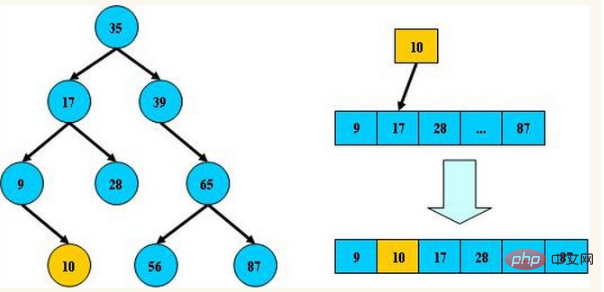平衡树的层级结构：因为平衡二叉树查询性能和树的层级(h高度)成反比，h值越小查询越快、为了保证树的结构左右两端数据大致平衡降低二叉树的查询难度一般会采用一种算法机制实现节点数据结构的平衡，实现了这种算法的有比如Treap、红黑树，使用平衡二叉树能保证数据的左右两边的节点层级相差不会大于1.，通过这样避免树形结构由于删除增加变成线性链表影响查询效率，保证数据平衡的情况下查找数据的速度近于二分法查找。
更多相关知识，请访问 PHP中文网！！

展开全文• 一、什么是平衡二叉树平衡二叉树(Self-Balancing Binary Search Tree 或者 Height-Balancing Binary Search Tree)译为 自平衡的二叉查找树或者高度平衡的二叉查找树，简称平衡二叉树，也叫 AVL 树，是一种二叉排序树......